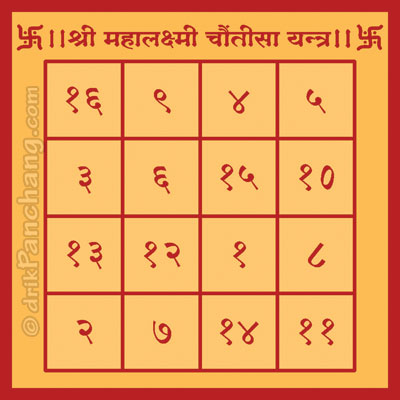Useful Tips on
Panchang
Lakshmi Chautisa Yantra
Lakshmi Chautisa YantraLakshmi Chautisa Yantra
Lakshmi Chauntisa YantraLakshmi Chauntisa Yantra
In Yantra Sadhana, Chauntisa Yantra is considered very powerful and it is considered the embodiment of happiness and prosperity. One of the Chauntisa Yantra is dedicated to Goddess Lakshmi and popularly known as Lakshmi Chauntisa Yantra.

Lakshmi Chauntisa Yantra is drawn on Bhoja Patra with red ink made of sandalwood paste and with pen made of pomegranate tree. The Yantra is kept in front of the Goddess Lakshmi during Diwali Puja. On the next day Yantra is put in the office or at home where money is kept. This remedy brings wealth and prosperity to business and home.

Apart from Diwali Lakshmi Puja, the Yantra can also be worshipped during Ravi Pushya Nakshatra day.

Significance of Chauntisa Yantra
In Devanagari, Chauntisa represents the number 34. As the numbers drawn on Yantra grid sum to 34 in various permutations and combinations, the Yantra is known as Chauntisa or Chautisa i.e. the one which counts to 34.

It is interesting to note that all 4 rows, 4 columns, 2 diagonals, all 2x2 sub-squares, the corners of each 3x3 squares and 4x4 squares, corners of 3 vertical and 3 horizontal rectangles, corners of 2 inclined rectangles sum to 34.

Rows of Chauntisa Yantra
1. 16 + 09 + 04 + 05 = 34
2. 03 + 06 + 15 + 10 = 34
3. 13 + 12 + 01 + 08 = 34
4. 02 + 07 + 14 + 11 = 34
Columns of Chauntisa Yantra
1. 16 + 03 + 13 + 02 = 34
2. 09 + 06 + 12 + 07 = 34
3. 04 + 15 + 01 + 14 = 34
4. 05 + 10 + 08 + 11 = 34
Diagonals of Chauntisa Yantra
1. 16 + 06 + 01 + 11 = 34
2. 05 + 15 + 12 + 02 = 34
Corners of 2x2 sub-squares
1. 16 + 09 + 06 + 03 = 34
2. 09 + 04 + 15 + 06 = 34
3. 04 + 05 + 10 + 15 = 34
4. 03 + 06 + 12 + 13 = 34
5. 06 + 15 + 01 + 12 = 34
6. 15 + 10 + 08 + 01 = 34
7. 13 + 12 + 07 + 02 = 34
8. 12 + 01 + 14 + 07 = 34
9. 01 + 08 + 11 + 14 = 34
Corners of 3x3 sub-squares
1. 16 + 04 + 01 + 13 = 34
2. 09 + 05 + 08 + 12 = 34
3. 03 + 15 + 14 + 02 = 34
4. 06 + 10 + 11 + 07 = 34
Corners of the 4x4 full-square
1. 16 + 05 + 11 + 02 = 34
Corners of vertical rectangles
1. 16 + 09 + 07 + 02 = 34
2. 09 + 04 + 14 + 07 = 34
3. 04 + 05 + 11 + 14 = 34
Corners of horizontal rectangles
1. 16 + 05 + 10 + 03 = 34
2. 03 + 10 + 08 + 13 = 34
3. 13 + 08 + 11 + 02 = 34
Corners of inclined rectangles
1. 04 + 10 + 07 + 13 = 34
2. 03 + 09 + 08 + 14 = 34
10.160.15.202